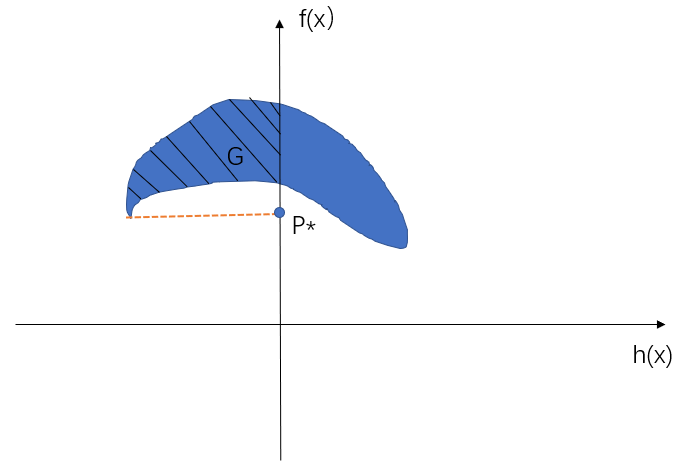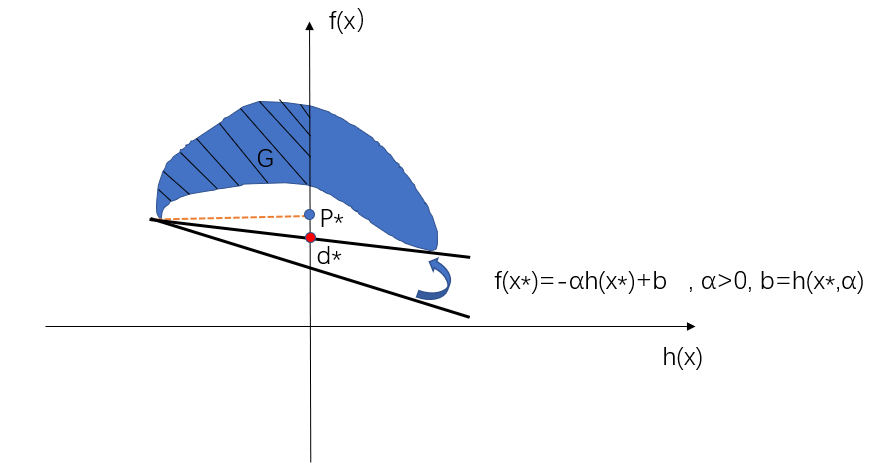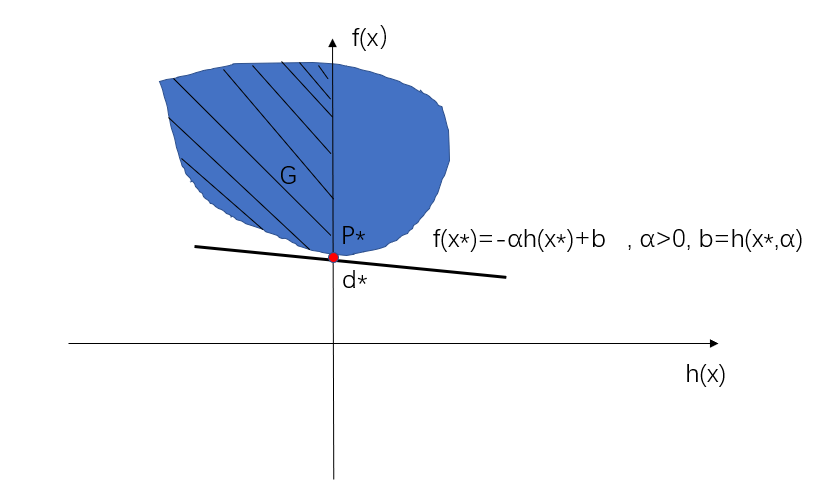• 约束优化、拉格朗日对偶问题
千次阅读
2019-01-27 17:08:50

## 优化问题分类

### 根据约束条件分类

约束问题分为无约束优化问题、等式约束优化问题、不等式约束优化问题三类。

### 根据优化函数和约束条件分类

线性规划问题：优化函数线性，约束函数线性；
二次规划问题：优化函数二次，约束函数线性；
非线性规划问题：优化函数非线性，约束函数非线性。

## 无约束优化

例如求 min ⁡ f ( x , y ) \min f(x,y) ，没有其它的等式或者不等式约束，充其量有定义域的限制。

### 求解方法

根据Fermat定理，求导，若无解析解，可借助梯度下降法、牛顿法等。

## 等式约束优化

在无约束优化问题的基础上，加了一些等式约束条件。举例：
min ⁡ f ( x , y ) s . t . ( s u b j e c t   t o )         h i ( x , y ) = 0 , i = 1 , 2 … \begin{aligned} &amp; \min f(x,y)\\ &amp; s.t. (subject~to)~~~~~~~h_{i}(x,y)=0, i=1,2 \dots \end{aligned}
除了最基本的目标函数外，还有若干等式约束条件。

### 求解方法

利用拉格朗日乘数法，构造拉格朗日函数。
L ( x , y , α ) = f ( x , y ) + ∑ α i h i ( x , y ) L(x,y,\alpha)=f(x,y)+\sum \alpha_{i}h_{i}(x,y)
对自变量x,y和拉格朗日乘子 α \alpha 求偏导，结果等于0，构造i+2个方程
{ ▽ x , y L = 0 ▽ α L = 0 \left\{ \begin{aligned} \bigtriangledown _{x,y} L &amp; = 0 \\ \bigtriangledown _{\alpha } L &amp; = 0 \end{aligned} \right.
解得 x , y , α x,y,\alpha 的值后，把 x , y x,y 带回验证，即可求得。
几何意义： f ( x , y ) f(x,y) 在x-y平面的等高线与 h i ( x , y ) h_{i}(x,y) 相切，法向量平行，梯度相等，此时 α \alpha 是个标量，不改变方向。具体的几何意义，可以看blog：https://www.cnblogs.com/ooon/p/5721119.html 或者直接百度百科"朗格朗日乘数法"。

## 不等式约束优化

在等式约束优化问题的基础上，加了若干不等式约束条件。例如：
m i n     f ( x , y ) s . t .     h i ( x , y ) = 0 , i = 1 , 2 … g j ( x , y ) ≤ 0 , j = 1 , 2 … \begin{aligned} \ min~~~&amp;f(x,y) \\ s.t.~~~&amp;h_{i}(x,y)=0,i=1,2 \dots\\ &amp;g_{j}(x,y) \leq 0,j=1,2 \dots \end{aligned}
除了最基本的目标函数，若干等式约束条件外，又加了若干不等式约束条件。注意不等式约束条件如果不是小于等于0的形式，就要化为这样的标准形式。

### 求解方法

利用拉格朗日乘数法+KKT条件，构造拉格朗日函数如下：
L ( x , y , α , β ) = f ( x , y ) + ∑ α i h i ( x , y ) + ∑ β j g j ( x , y ) L(x,y,\alpha,\beta)=f(x,y)+\sum \alpha_{i}h_{i}(x,y)+\sum \beta_{j}g_{j}(x,y)
KKT条件如下：
(1) ▽ x , y L ( x , y , α , β ) = 0 \bigtriangledown_{x,y} L(x,y,\alpha,\beta)=0 \tag{1}

(2) h i ( x , y ) = 0 h_{i}(x,y)=0 \tag{2}

(3) g j ( x , y ) ≤ 0 g_{j}(x,y) \leq 0 \tag{3}

(4) β j ≥ 0 \beta_{j}\geq 0 \tag{4}

(5) β j g j ( x , y ) = 0 \beta_{j}g_{j}(x,y)=0 \tag{5}

(6) α i ≠ 0 \alpha_{i} \neq 0 \tag{6}

其中 ( 2 ) ( 3 ) (2)(3) 不用解释，初始约束条件， ( 4 ) (4) 是不等式约束拉格朗日乘子需要满足的条件， ( 5 ) (5) 松弛互补条件， ( 6 ) (6) 是等式约束拉格朗日乘子需要满足的条件。对于这几个条件的理解，可以看blog：https://www.cnblogs.com/ooon/p/5721119.html

问题：如果求的是最大值 max ⁡ f ( x , y ) \max f(x,y) ，KKT条件是否还长这样？一些KKT条件的符号会不会变？

### 拉格朗日对偶问题

原始问题：先固定自变量 x , y x,y ，求拉格朗日乘子 α , β \alpha,\beta 使得 L ( x , y , α , β ) L(x,y,\alpha,\beta) 最大，再求自变量 x , y x,y 使得 L ( x , y , α , β ) L(x,y,\alpha,\beta) 最小。
min ⁡ x , y θ P ( x , y ) = min ⁡ x , y max ⁡ α , β L ( x , y , α , β ) \min_{x,y}\theta_{P}(x,y)=\min_{x,y}\max_{\alpha,\beta} L(x,y,\alpha,\beta)
对偶问题：先固定拉格朗日乘子 α , β \alpha,\beta ，求自变量 x , y x,y 使得 L ( x , y , α , β ) L(x,y,\alpha,\beta) 最小，再求拉格朗日乘子 α , β \alpha,\beta 使得 L ( x , y , α , β ) L(x,y,\alpha,\beta) 最大。
max ⁡ α , β θ D ( x , y ) = max ⁡ α , β min ⁡ x , y L ( x , y , α , β ) \max_{\alpha,\beta}\theta_{D}(x,y)=\max_{\alpha,\beta} \min_{x,y} L(x,y,\alpha,\beta)
可以证明，原始问题和优化问题完全一样，想让对偶问题与优化问题完全一样，需要满足强对偶性。具体可以看blog:https://www.cnblogs.com/ooon/p/5723725.html 。下面写一下重点。
弱对偶性： max ⁡ α , β θ D ( x , y ) ≤ min ⁡ x , y θ P ( x , y ) \max_{\alpha,\beta}\theta_{D}(x,y) \leq \min_{x,y}\theta_{P}(x,y) ；强对偶性： max ⁡ α , β θ D ( x , y ) = min ⁡ x , y θ P ( x , y ) \max_{\alpha,\beta}\theta_{D}(x,y) = \min_{x,y}\theta_{P}(x,y)
其中弱对偶性升级为强对偶性需要满足：slater条件或者KKT条件。李航在《统计学习方法》书中，提及了强对偶性成立的一种情况：当 f ( x ) f(x) g ( x ) g(x) 为凸函数， h ( x ) h(x) 为仿射函数， g ( x ) g(x) 严格可行。拉格朗日乘数法
更多相关内容
• 本文主要探讨优化问题中强、弱对偶性以及KKT条件的证明。

# 1.原问题

首先给出问题的一般形式：上式表明我们一共有M+N个约束条件，对于不是求最小值或者约束条件大于等于0的情况，我们添加一个负号就可以变成上面这种形式。
上述问题我们一般称之为带约束的原问题。

利用拉格朗日乘子法，我们构造一个新的函数以及约束条件如下：其中：我们称上面的问题为无约束的原问题（对x不再有约束）。上述L是拉格朗日乘子法的基本形式，这个就不再证明。

# 2.对偶问题

对于无约束的原问题，我们先直接给出它的对偶问题形式（其实就是简单交换min和max）：上述问题我们称之为原问题的对偶问题。

## 2.1弱对偶性的一般证明

所谓弱对偶性，指的是：在再谈SVM（hard-margin和soft-margin详细推导、KKT条件、核技巧）中，我们大致口头证明了弱对偶性的成立，即“凤尾”>=“鸡头”。何谓“凤尾”？我先选出最强的一批人( max ⁡ f \max f )，然后组成实验班，实验班倒数第一就是 min ⁡   max ⁡ f \min \ \max f ；何谓“鸡头”？我先选出最弱的一批人( min ⁡ f \min f )，然后在这批比较弱的人当中选出最强的那个人，也即是 max ⁡   min ⁡ f \max \ \min f ，那么“鸡头”与“凤尾”孰强孰弱，是显而易见的。
现在我们利用数学推导来大致证明一下弱对偶性。
对于 L ( x , λ , η ) L(x,\lambda,\eta) 这个函数，我们知道下面这个不等式一定成立：上面这个不等式很好理解，中间 L ( x , λ , η ) L(x,\lambda,\eta) 我们可以理解为L的值域，值域里面的任何一个数，必然是大于等于它的最小值，小于等于它的最大值。其实这一步已经证明出弱对偶性了，不过为了更容易理解，我们可以进一步说明。
上述不等式最左边的表达式最后是关于 λ , η \lambda,\eta 的一个函数，而最右边是一个关于 x x 的函数，因此我们又令：因此我们有：证毕。

## 2.2弱对偶性的几何证明

为了使问题简化，同时方便证明，我们去掉原问题中等式的约束条件，同时不等式约束条件只保留一个，即原问题变成：那么拉格朗日函数就变成：我们又令：p ∗ p^* 是原问题的最优解， d ∗ d^* 是对偶问题的最优解。证明弱对偶性实际上就是证明 d ∗ ≤ p ∗ d^*\leq p^*
我们令区域G的表达形式为：D是原问题的定义域，G表示一个个点的集合，点的横坐标是约束条件 u = m 1 ( x ) u=m_{1}(x) ，纵坐标是原函数 t = f ( x ) t=f(x)
有了上述集合G的定义之后，我们就可以对 p ∗ , d ∗ p^*,d^* 进行变式。
首先对 p ∗ p^* 进行变式：因为 t = f ( x ) t=f(x) ，所以 p ∗ p^* 实际上就是t的最小值，反映到集合G中去就是指一个点的纵坐标，这个点要满足两个条件：一是肯定要在G中，二是 m 1 ( x ) ≤ 0 m_{1}(x)\leq0 也就是该点的横坐标小于等于0。
如下图所示：我们对 u ≤ 0 u\leq0 那部分，也就是图中阴影部分上的每个点，找到一个最低的点，它的纵坐标就是 p ∗ p^*
接着对 d ∗ d^* 进行变形：上述 t + λ u t+\lambda u 的来源为：对变形后 d ∗ d^* 我们令：我们先找到 g ( λ ) g(\lambda) 在图中的位置：我们知道 t + λ u t+\lambda u 实际上也是一个值，我们不妨令 t + λ u = k t+\lambda u=k ，该式表示一条斜率为 − λ -\lambda 并过(0,k)的直线，我们要找的是 t + λ u t+\lambda u 的最小值，实际上就是k的最小值，实际上就是该直线与纵轴交点的最小值。而在求 min ⁡ x   t + λ u \min \limits_{x}\ t+\lambda u 的最小值时， λ \lambda 固定的，因此斜率 − λ -\lambda 也是固定的：我们保持斜率不变移动直线，不断往上移动，则该直线与纵轴交点的纵坐标k也不断增大，因为限制条件还有一个就是 ( u , t ) ∈ G (u,t)\in G ，因此该直线必须经过区域G，我们一直往上移动，直到直线第一次与G相交，记下相应的k值为 k 1 k_{1} ，再继续往上也都满足条件，知道该直线与G不再相交，但是现在我们求得是最小值，那么最小值其实就是 k 1 k_{1} ，即 g ( λ ) g(\lambda) 就等于 k 1 k_{1}
进一步，我们要求：这里要重点注意：上一步我们求得了 g ( λ ) g(\lambda) 就等于 k 1 k_{1} ，但是这种情况只是一种情况，在上一步求 g ( λ ) g(\lambda) 时，我们假若改变斜率 − λ -\lambda ，那么 k 1 k_{1} 的值是会变的，如下所示：我们换一个 λ \lambda 固定时， g ( λ ) g(\lambda) 也就是 k 1 k_{1} 自然也就在变。
第二步要干的其实就是让我们求这个 g ( λ ) g(\lambda) 的最大值。那什么时候是最大的？实际上就是以G的最低点为轴，我们旋转直线，直到与左上方最低点相交时， g ( λ ) g(\lambda) 是最大的。如下所示：可能很多人就有疑问了：我为什么不可以让斜率继续增大？让直线穿过G？这里有疑问的同学不妨回忆一下第一个步骤：我们在确定 g ( λ ) g(\lambda) 的时候，第一次相切我们就停止了，而后改变斜率继续相切：如上图所示，假如你继续增大斜率，那么该直线就不跟G的最低点相切了。我们是先推第一步再推第二步，而推第二步的时候肯定必须满足第一步的条件，所以 d ∗ d^* 只能是在那个位置。从上图也可以看出来： d ∗ ≤ p ∗ d^*\leq p^* ，也就是对偶问题的解是小于等于原问题的解的，也即是说满足弱对偶性。因此这里我们进一步证明了弱对偶性。

## 2.3强对偶性的几何表示以及条件

什么是强对偶性？就是指原问题的解与对偶问题的解是相同的，也即是： d ∗ = p ∗ d^*=p^*
画个图：假设G是一个凸集，那么根据上面找 d ∗ d^* p ∗ p^* 的思路，我们很容易知道这个时候二者是相等的，也就是满足强对偶关系。
那上面这句话的意思就是说：只要是凸集就一定满足强对偶关系。这句话不是正确的，不是所有的凸集都满足强对偶关系，但是加上slater条件就一定满足。

## 2.4 slater condition

先直接给出slater条件的定义：对于x的定义域D，如果它存在一个内点（不是边界上的点） x ∗ x^* 满足对任意的 m i ( x ) < 0 , i = 1 , 2 , . . . , M m_{i}(x)\lt0,i=1,2,...,M ，则说明该问题满足slater条件。
对slater条件做两点说明：

1. 对于大多数的凸优化问题来说，slater condition都是成立的
2. 放松的slater条件：如果约束函数 m i ( x ) , i = 1 , 2 , . . . , M m_{i}(x),i=1,2,...,M 中有K个是仿射函数，则我们只需要那M-K个约束函数满足第一个条件，我们也说该问题满足slater条件。
3. 什么是仿射函数？仿射函数，即最高次数为1的多项式函数。常数项为零的仿射函数称为线性函数。简单来说，就是比较简单的函数。

对第一个条件进一步说明：为什么我们要满足这个条件？第一个条件放在G中的意思就是：G在u<0部分必须有点存在假设纵坐标左边没有点，那么在我们寻找 g ( λ ) g(\lambda) 时，那条直线实际上就会是纵坐标轴。

# 3.KKT条件的证明

通过上面的推导我们知道了：满足强对偶关系之后我们就得到一个结论： d ∗ = p ∗ d^*=p^* ，但是也到此为止了，我们肯定得解出那些未知最优参数（带 * 的变量），KKT条件就是干这件事。
KKT条件有三部分：可行条件、互补松弛条件以及偏导为0条件，我们一个一个推导。

## 3.1可行条件

所谓可行条件，指的是一开始就满足的一些条件：这三个条件肯定得满足，这个没啥可说的，天然满足

## 3.2互补松弛条件

我们知道：带星号的都是最优解的意思。根据可行条件我们知道： λ i ≥ 0 , m i ≤ 0 \lambda_{i}\geq0,m_{i}\leq0 ，所以 λ i m i ≤ 0 \lambda_{i}m_{i}\leq0 ，所以上面继续变换：因为最后一步等于第一步，所以中间推导步骤中的 ≤ \leq 都应该变成=，倒数第三步等于倒数第二步，所以我们有：而前面我们又知道 λ i m i ≤ 0 \lambda_{i}m_{i}\leq0 ，所以互补松弛条件如下：## 3.3偏导为0条件继续看这个推导，第二、三步之间应该用等号连接，即：意思就是说L在 x = x ∗ x=x^* 处有最小值，于是偏导为0条件就出来了：于是KKT条件为：证毕。

欢迎大家关注我的微信公众号：KI的算法杂记，有什么问题可以直接发私信。展开全文凸函数
• 咨询下各位，在机器学习相关内容中，每次看到带约束优化问题，总是看到先用拉格朗日函数变成无约束问题，然后转成求拉格朗日对偶问题，然后有凸函数假设，满足KKT条件时原问题最优解和对偶问题最优解等价。...

# 咨询：带约束优化问题 拉格朗日 对偶问题 KKT条件

咨询下各位，在机器学习相关内容中，每次看到带约束优化问题，总是看到先用拉格朗日函数变成无约束问题，然后转成求拉格朗日对偶问题，然后有凸函数假设，满足KKT条件时原问题最优解和对偶问题最优解等价。

每次看到这个，总不是很理解为什么要这么做？
为什么首先转为无约束问题（这个相对好理解一点，因为容易处理）
为什么拉格朗日函数无约束问题要转变成求拉格朗日对偶问题求解？
如果一开始的约束问题f(x)不是凸函数，那怎么办？

忘各位了解的可以将这里的流程和原理可以详细解答一下，看过前面有些人解答问题将问题的前世今生都讲解一遍，很清晰。这个问题一直都让我有点混乱，希望能在各位帮助下了解更清楚

补充一个：带惩罚的优化问题是不是跟这一样是通过求对偶问题还求解的
看你的问题，我猜测你应该是在看SVM，关于SVM这东西，我认为它可以分成三个独立的成分：
1.最优分离超平面
2. kernel映射
3. 拉格朗日对偶
这三个部分中的每一个都有一套理论，最优分离超平面就不说了，这就是SVM的根基，kernel并不是SVM独有的（只是在SVM里比较出名），kernel有一套核方法，主要是为了解决映射到高维空间后引起的维数灾难问题。我们知道，SVM只靠最优分离超平面的话只能实现线性分割，而使用了kernel映射后就可以实现非线性分割了，在这个转换过程中，拉格朗日对偶起了中间桥梁的作用。拉格朗日对偶也不是SVM特有的，它属于凸优化的内容。在SVM的很多教程中都跳过了拉格朗日对偶的讲解，下面我们将进一步讨论拉格朗日对偶这个问题（并不完全讲，也只是讲个大概）

在此之前，我们要再重申一遍什么是凸函数

跟我念：凸凸凸凸凸凸凸凸凸凸凸凸凸凸
（威廉王子：我都躺这么远了还TM中枪？！）

此外，我们还需要讲清楚一些前置内容，首先：什么是优化问题？
所谓优化问题，也就是要实现
minf0(x)
s.t.fi(x)bi,i=1,,m
fi(αx+βy)αfi(x)+βfi(y)
fi(αx+βy)=αfi(x)+βfi(y)

# ==================================================

f(x)=sup{g(x)|g仿射，且z,g(x)f(z)}
f(x)=max{f1(x),f2(x),}
g(x)=supyAf(x,y)

# ==================================================

minf0(x)
s.t.fi(x)0,i=1,,m
hi(x)=0,i=1,,p
L(x,λ,μ)=f0(x)+i=0mλifi(x)+i=1pμihi(x)
g(λ,μ)=infxDL(x,λ,μ)=infxDf0(x)+i=0mλifi(x)+i=1pμihi(x)
i=0mλifi(x)+i=1pμihi(x)
L(x^,λ,μ)=f0(x^)+i=0mλifi(x^)+i=1pμihi(x^)f0(x^)
g(λ,μ)=infxDL(x,λ,μ)L(x^,λ,μ)f0(x^)
minxTx
s.t.Ax=b
L(x,μ)=xTx+μT(Axb)
ΔxL(x,μ)=2x+ATμ=0
x=12ATμ
g(μ)=infxL(x,μ)
g(μ)=infxL(12ATμ,μ)=14μTAATμbTμ
14μTAATμbTμinf{xTx|Ax=b}
minCTx
s.t.Ax=b
x0
L(x,λ,μ)=CTxi=1nλixi+μT(Axb)=bTμ+(C+ATμλ)Tx
g(λ,μ)=infxL(x,λ,μ)=bTμ+infx(C+ATμλ)Tx
g(λ,μ)=bTμ,        如果ATμλ+c=0
g(λ,μ)=,        其他
maxbTμ
s.t.ATμλ+c=0
λ0
maxbTμ
s.t.ATμ+c0
maxCTx
s.t.ATxb
L(x,λ)=CTx+λT(Axb)=bTλ+(ATλ+C)Tx
g(λ)=infxL(x,λ)=bTλ+infx(ATλ+C)Tx
g(λ)=bTλ,        如果ATλ+c=0
g(λ)=,        其他
maxbTλ
s.t.ATλ+c=0
λ0
展开全文• SVM中拉格朗日乘子法、KKT条件对偶问题详解创作目的1.SVM回顾2.拉格朗日乘子法3.KKT条件4.对偶问题对偶性证明总结 创作目的 我是机器学习初学者，目前正在上机器学习课，老师的SVM部分讲解的非常细致，我收获颇...

# 创作目的

我是机器学习初学者，目前正在上机器学习课，老师的SVM部分讲解的非常细致，我收获颇丰于是想自己记录一下SVM的详细内容以及推导过程方便自己以后复习。这一节主要针对拉格朗日乘子法以及其KKT条件还有强对偶、弱对偶问题进行总结和推导，希望在这一块有疑问的同学可以一起交流学习。萌新第一次写博客，很多地方表述不足希望大家指出。

## 1.SVM回顾

支持向量机（SVM）是目前最好的有监督分类方法之一，SVM分类器的基本型的推导很多书以及网上都有这里就不再介绍了，如果有时间以后可以补上。知乎上我找到一位作者总结的很到位感兴趣的读者可以去看看：支持向量机基本概念

SVM基本型基于两类最大化间隔的思想等价于下面这个优化问题：（1） 式本身就是一个凸二次规划问题，凸函数以及凸二次规划的定义可以见链接：

https://blog.csdn.net/promisejia/article/details/81241201

凸二次规划问题可以直接用现成的优化计算包求解但这样计算较复杂，所以我们引入朗格朗日乘子法对其"对偶问题"进行更高效的求解。（1）的对偶问题为：上式必须要满足KKT条件：将KKT条件最后两项代入约束目标，这样原问题（1）就转换成了只有α的二次规划问题，一般采用SMO算法可以很快求解出近似解，SMO算法我将在下一次详细总结。

身边很多同学刚开始学SVM到这的时候就开始“黑人问号.jpg”了。什么是拉格朗日乘子？什么是对偶问题？为什么求min就转变成求max min了？KKT条件又是什么鬼？下面我一一进行解答。

## 2.拉格朗日乘子法

拉格朗日乘子法最开始是只针对等式约束优化问题提出的。对于一个等式约束优化问题：引入一个自变量α，α可以取任意值，则（2）严格等价于：现在来证明一下，我们把（3）式分满足（2）的约束条件和不满足两种情况讨论，即h(x)=0和h(x)≠0：（4）与（3）等价，同时对于（4）由于α可以取任意值，当h(x)≠0时，αh(x)可以无限大，那么取最小值时永远不可能取到h(x)≠0的情况。那么（3）就严格等价于h(x)=0的情况，也就是（2）了，证毕。

但是SVM算法基本型是一个不等式约束优化，同样对于一个不等式约束：它等价于（6），这里α加上了约束，不再可以取任意数而是取大于等于0的数：证明同上，讨论（6）满足(5)的约束和不满足约束两种情况，即：对于（7）式第一排αh(x)小于等于0，对第一排求最大值时αh(x)=0，消掉后只留下f(x)。对于（7）第二排，αh(x)可以取到无限大所以取最小时不可能取到第二排，于是（6）就等价于（7）的第一排消掉αh(x)，也就是等价于（5），证毕。

同时我们看到（7）也等价于（5），我们把（7）表示成：通过（8）我们可以发现min max出现了 ，SVM基本型代入上式可以发现与SVM中的对偶问题只是在max min的顺序上不同了。这里强调一点，（8）式并不是（5）式的对偶问题，它们是严格等价的，是同一个问题，关于对偶问题我将在第四节细讲。下面来看满足（8）式的约束条件，也就是KKT条件。

## 3.KKT条件

刚才我们知道了（5）等价于（8），对于（8）最优解 x* 还应该满足以下的约束条件，统称为KKT条件：

a.首先，x* 要满足已有的两个基本约束，h(x*)<=0，以及 α>=0
b.同时（8）等价于（5）必须满足 αh(x*)=0 这个条件；
c.最后，我们在高中就学过对于一个无约束的凸函数求极小值问题的最优解x*一定满足 ∇ x f ( x ∗ ) = 0 \nabla_xf(x*)=0 这个条件，而当有约束条件时如（5）我们通过拉格朗日乘子法把（5）转化为（8）。（8）式先对max进行求极大值，再求min时，此时α的值已经固定，我们不妨将（8）约束目标min后边看成一个关于x的函数g(x)这时α不再构成约束,则（8）等价于对于无约束凸函数g(x)求极小值问题，所以一定满足条件： ∇ x f ( x ∗ ) + α ∇ x h ( x ∗ ) = 0 \nabla_xf(x*)+α∇xh(x*)=0
于是关于满足（8）的KKT条件就是：注意：KKT条件只是对于原问题的约束条件，最优解一定得满足KKT条件，KKT条件是原问题的充分必要条件。KKT条件与对偶问题无关只与原问题有关。

## 4.对偶问题

由前面分析我们知道了（5）等价于（8）。对于（8）式这样的不等式约束问题，我们可以定义其对偶问题。我们定义（8）的对偶问题为：可以看到这里的对偶问题仅仅知识交换了目标函数的max和min的求解顺序，约束条件都相同，所以原问题的约束条件KKT条件仍然是对偶问题的约束条件。对偶问题先确定里面x关于函数极小值有时候会比原问题先求α关于函数最大值更容易求解，比如SVM就是对偶问题比较好求解。但是对偶问题和原问题不是等价的，原问题和对偶问题有如下的关系：记f(x)+αh(x)为f(x,α)，上式推导过程：可以发现原问题目标函数始终是大于等于对偶问题的，所以我们定义当原问题目标函数的最优值等于对偶问题的值时称原问题满足强对偶性，大于时则为弱对偶的。只有当原问题有强对偶性我们才可以用对偶问题去等价原问题，那么什么时候原问题才满足强对偶性呢？答案是当原问题（5）是一个凸优化问题且满足salter条件时，它是强对偶的，下面来简单推导一下。

## 强对偶性证明

在B站上看见一位up讲解的特别好，这里再进行总结一下，附上链接：

https://www.bilibili.com/video/BV1aE411o7qd?t=427&p=33

对于一个约束优化问题，如（5）：他的原问题和对偶问题可以表示为：上一节提到原问题和对偶问题一定满足关系：p*>=d*，当"="成立时满足强对偶性。下面给出证明：
设D为f(x),h(x)的定义域的交集，为了方便表示出p和d的关系，我们将f(x)和h(x)表示为横纵坐标，建立一个平面直角坐标系，在定义域D下可以在该坐标系下定义原问题以及对偶问题的可行域G：为了便于理解，我们记h(x)轴为x轴，f(x)为y轴，（不失一般性）我们假设问题的可行域为一个非凸的区域,则该坐标系可以表示为：原问题最优解p*=min f(x)满足h(x)<=0，可行域即为上图阴影区域，要找此区域f(x)的极小值，即找到可行域中纵坐标最小的点即可，如上图蓝点位置就是该可行域的p*。

此外我们还需要找到d* 的值才能找到p* 和d* 的关系。我们把d*=max min f(x,α)拆成两部分，即:
第一部分：d*=max f(x*,α) (α是自变量，x* 是一个确定的数)；
第二部分：f(x*,α)=min f(x,α) ，(x是自变量，α是一个确定但未知的数)；
再由f(x,α)=f(x)+αh(x) 等价于 f(x)=-αh(x) + f(x,α),我们把d* 转化成了坐标系中的一次函数y=kx+b的形式。

首先，我们先看d* 的第二部分，它在坐标系中等价于f(x)=-αh(x) +b这条直线，由于α>0,所以h(x)的系数-α一定小于0，所以直线是经过二、四象限的，此外p* 一定在定义域G中所以直线与G必须要有交点才满足条件。第二部分的目标函数min f(x,α)即直线的常数项b，如下图，我们可以通过平移直线找到直线与可行域G相交时得到b的值，我们先固定一个α再将直线向上平移刚好与可行域相切时得到的常数项b最小。现在我们来看d* 的第一部分，也就是一次项系数-α的影响下常数项b求最大值，由于-α<0直线必须经过一、二、四象限，所以我们可以通过旋转直线求此时b的极大值。注意当直线与可行域相切时才满足第二部分的条件，如下图当直线旋转到非凸可行域两端点处相切时，既满足第一部分极小时的条件，又使得此时b最大，即此时对应的b就是d*。通过上图我们可以明显看到当可行域是非凸情形时d是小于p的，这也是弱对偶问题的另一种解释。那么当可行域是凸区域的情形会如何呢？我们看看下图：可以看到当可行域G为凸区域时，p* 和d* 刚好相等。目前已经证明：当原问题是一个凸优化问题，且满足slater条件时，可行域G就是一个凸区域，即原问题是强对偶问题。而SVM原问题是一个凸优化问题，同时是一个二次规划问题先天满足slater条件，所以SVM是一个强对偶性优化问题可以将原问题转换为对偶问题进行求解。

## 总结

本文主要对SVM问题中出现的拉格朗日乘子法以及其KKT条件还有强对偶、弱对偶问题进行总结和推导，用于个人期末复习以及加深印象。可能还有许多错误和不足，欢迎大家指正。下一节将对求解SVM对偶问题的主要算法SMO算法进行总结。

展开全文机器学习 svm 拉格朗日乘子法
• 拉格朗日乘数法、拉格朗日对偶问题的原理，拉格朗日对偶问题的充分条件和必要条件机器学习
• 主要介绍了约束优化问题中的KKT条件；拉格朗日对偶问题，包括原始问题对偶问题的关系、对偶上升法、对偶分解法等；内外点罚函数法，具体包括外点法和内点法。
• ## 对偶问题

千次阅读 2021-04-11 19:24:06
1.对偶问题 随着线性规划应用的逐步深入，人们发现个线性规划问题往往伴随着与之配对的、两者有着密切的联系的另个线性规划问题，将其中个称为原问题，另外个称之为对偶问题对偶理论深刻揭示了原问题与...线性规划 机器学习 支持向量机
• 对偶理论 、 1、对称性定理 、 2、弱对偶定理 、 3、最优性定理 、 4、强对偶性 、 5、互补松弛定理 、 二、原问题对偶问题对应关系 、 二、对偶理论的相关结论 、 1对偶问题存在 、 2、对偶问题...最优性定理
• ## 对偶问题的转换

万次阅读 2019-07-21 10:57:32
对偶问题 下面是博主对对偶问题的一些个人理解，博主也是小白个，...那么对于途径1，小明同学想要在有限的生产资源约束下，最大化自身的利润,这就是原问题。 对于途径2，小明同学作为工厂的拥有者，他所能接受的...
• ## 线性规划技巧: 如何写对偶问题

千次阅读 多人点赞 2020-03-19 22:37:19
给定线性规划的原始问题,...原问题约束对应对偶变量. 对偶变量乘以原问题约束的右端(bbb)得到对偶问题的目标. 原问题目标的系数ccc对应对偶问题约束的右端. 最小化对应最大化(反之亦然). 如果原问题有等式...算法
• 第一篇文章主要介绍了如何从拉格朗日函数出发，构造对偶问题，并阐述强对偶性与KKT条件的关系；第二篇文章可以视作对偶问题理论在线性规划里的应用。 拉格朗日函数和对偶问题 优化问题一般都能转化为如下的形式： ...
• ## 对偶问题的理解

千次阅读 2018-08-08 16:16:54
1. 为什么要使用对偶问题（SVM） 1. 对偶问题将原始问题中的约束转为了对偶问题中的等式约束 2. 方便核函数的引入 3. 改变了问题的复杂度。由求特征向量w转化为求比例系数a，在原始问题下，求解的复杂度与...SVM
• 任一线性规划问题都存在另与之伴随的线性规划问题，它们组成一对互为对偶的线性规划问题。 线性规划的对偶问题与原问题互为对偶，线性规划的原问题对偶问题地位具有对称关系。 2. 原问题对偶问题的对应关系 3...线性规划
• 之所以在线性回归之后写SVM，是因为LogisticRegression可以认为是通过单调可微函数----Sigmod函数将回归问题引申为分类问题；而SVM则可以看做使用线性回归模型以及到所确定...先定义原始问题的拉格朗日“对偶函数”...机器学习 SVM
• ## 线性规划对偶问题

万次阅读 多人点赞 2021-04-28 23:49:31
线性规划对偶问题线性规划及单纯形法〇． 前言对偶问题的提出二． 对称形式下对偶问题的一般形式三． 非对称形式的原-对偶问题关系四． 对偶问题的基本性质五． 对偶问题的单纯形法描述六． 影子价格七． 利用...
• 本篇是写在SVM之前的关于优化问题的一点知识，在SVM中会用到。考虑到SVM之复杂，将其中优化方面基础知识提出，单作此篇。所以，本文也不会涉及优化问题的许多深层...对于个含约束的优化问题： {minxf(x)s.t.x∈C机器学习
• 标准型的对偶问题 线性规划的标准型如下： 目标函数：max   c1x1+c2x2+...+cnxn等式约束：P1x1+P2x2+...+Pnxn=b⃗非负性约束：xj≥0          &...机器学习 人工智能
• ## 数学之对偶问题

千次阅读 2017-09-06 22:02:16
线性规划的对偶问题常山机器厂生产Ⅰ、Ⅱ两种产品。这两种产品都要分别在A、B、C三种不同设备上加工。按工艺资料规定，生产每件产品Ⅰ需占用各设备分别为2h、4h、0h，生产每件产品Ⅱ需占用各设备分别为2h、0h、5h，...
• 上一节，我们介绍了slater条件，假如说我们遇到个凸优化问题+slater条件，那么一定满足强对偶关系。强对偶关系是说p*是原问题的解，d*是对偶函数的解，满足p*=d*。p*对应最优解是x*，d*对应最优解是λ*和η*。...
• 最近看论文里面有好多凸优化、对偶函数、KKT什么的，太顶了，看不下去，就先补补这方面的知识。 凸优化其实是个比较大的问题，看了半天也是一知半解，只能理解大概的原理和意义，这里就先记录一下，后面有更深的...机器学习
• 上面我们讲到了怎么对硬间隔SVM进行求解，我们我们先把带约束问题，转化为无约束问题，通过强对偶关系将minmax转为maxmin，对w,b求min，最终我们求出来最小值，然后关于λ求最大值。 我们最后圈出来的地方，...
• 1）解释如何判断问题是不是线性规 （2）讲解如何构造个线性规划的对偶问题 （3）列举出关于个线性规划和它的对偶问题的基础结论。 这篇笔记不提供任何证明过程，也不解释任何线性规划对偶性中隐含的深层...
• 目录原问题的转化对偶问题 ...关于对偶问题的动机说完了，下面我们把注意力集中在minf(x)min f(x)minf(x)上，然后有两条约束条件，其实约束条件相当于将解空间划分为了两块，空间1空间1空间1是符合约束条件，空间2空算法
• 每个线性规划问题都有个与之对应的对偶问题对偶问题是以原问题约束条件和目标函数为基础构造而来的。对偶问题也是个线性规划问题，因此也可以采用单纯形法进行求解. 然而，接下来将会发现，对偶问题的最优解...优化
• 、互补松弛定理作用、 二、影子价格、 三、影子价格示例、
• 最近学习SVM的相关内容时，接触到了拉格朗日函数及其对偶问题，于是就学习了一些相关内容，在此整理总结一下。 文章目录1....但是对于有约束条件的求函数极值的问题该怎么处理？ 定义：称满足以下条件的(x0,拉格朗日函数
• 每个线性规划问题都有个与之对应的对偶问题对偶问题是以原问题约束条件和目标函数为基础构造而来的，在求出问题解的时候，也同时给出了另一问题的解。在某些情况下，利用对偶理论求解线性规划问题会更为...机器学习 算法
• 给定个二分类问题的训练样本集D={(x1,y1),(x2,y2),...,(xm,ym)},yi∈{−1,+1}D={(x1,y1),(x2,y2),...,(xm,ym)},yi∈{−1,+1}D=\{(\boldsymbol{x}_1,y_1),(\boldsymbol{x}_2,y_2),...,(\boldsymbol{x}_m,y_m)\},y_i...SVM 推导...